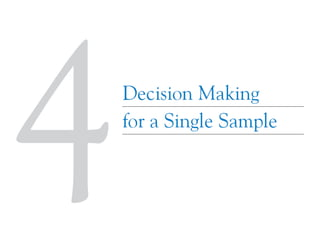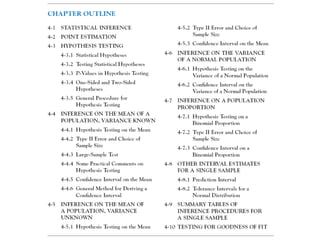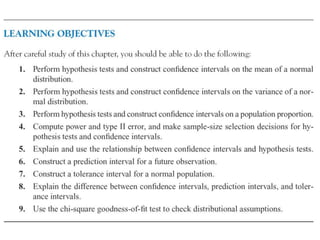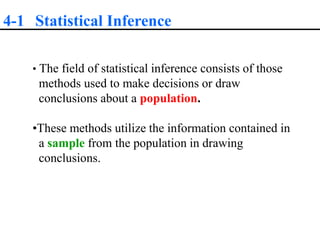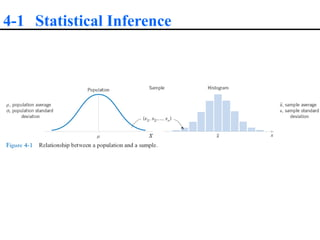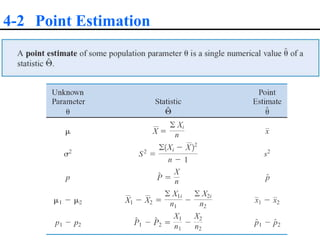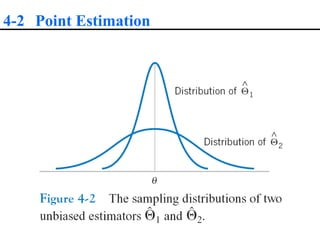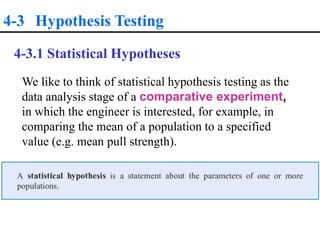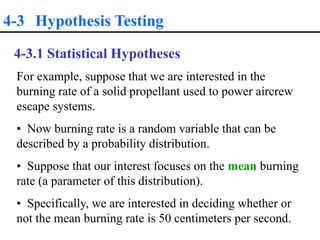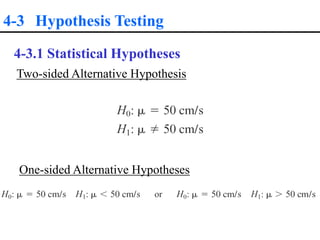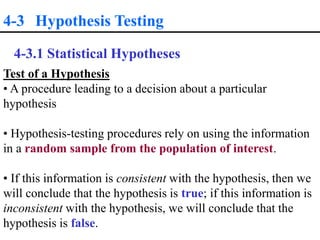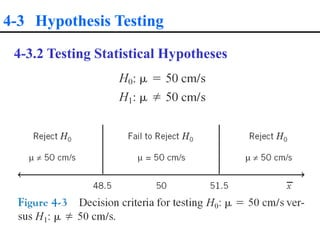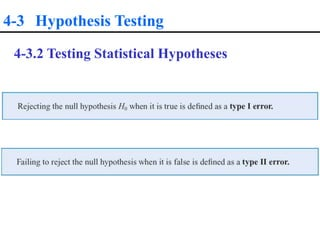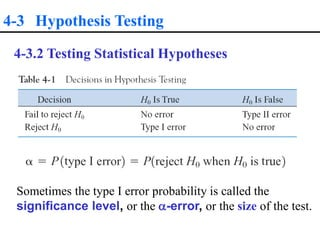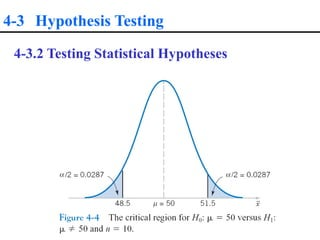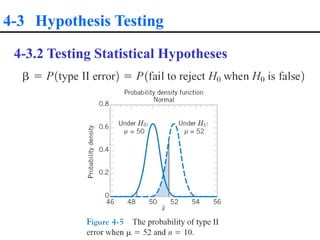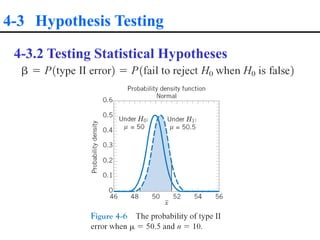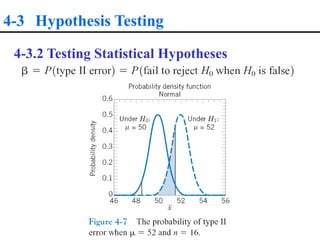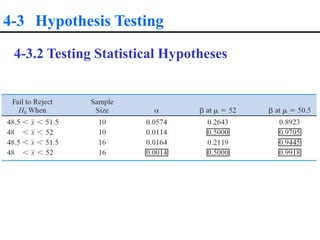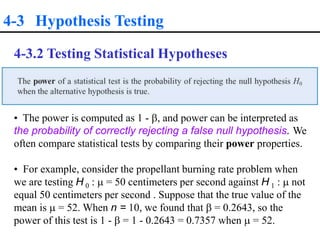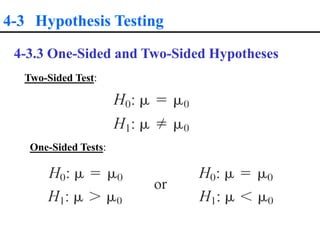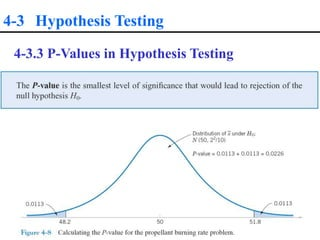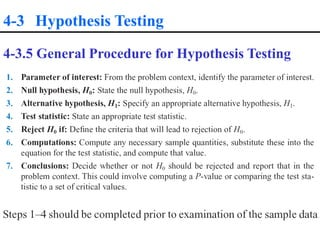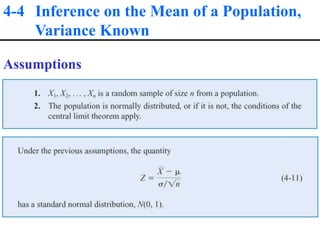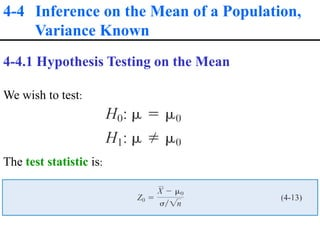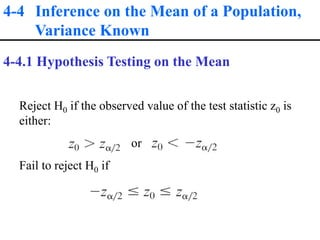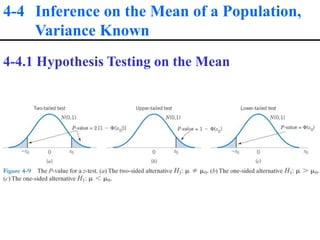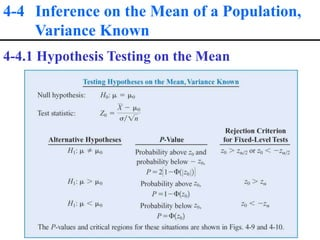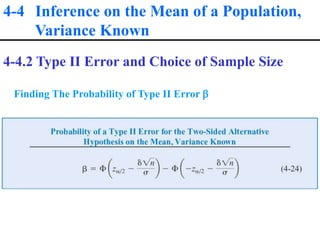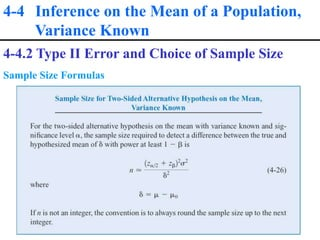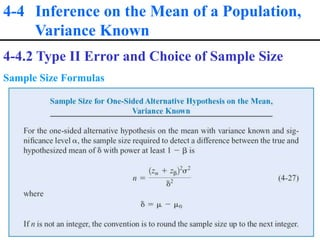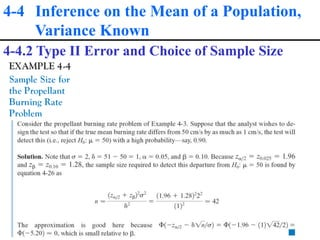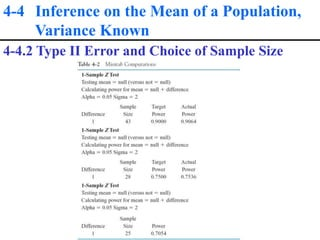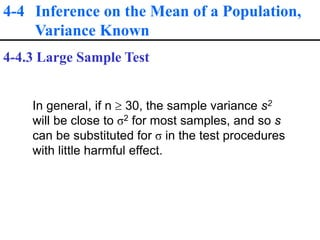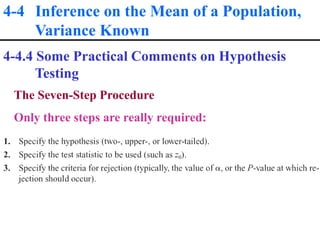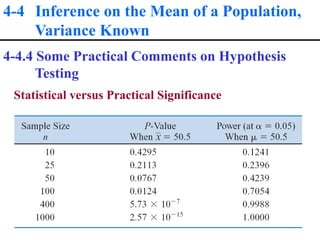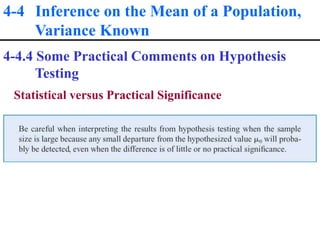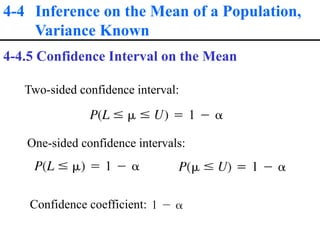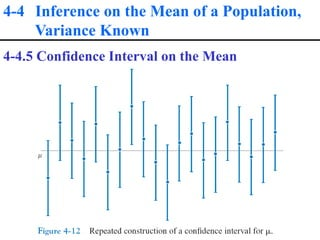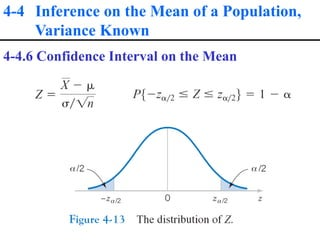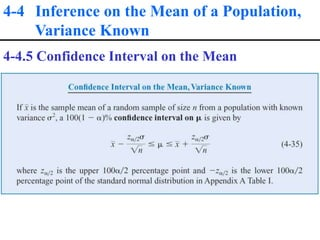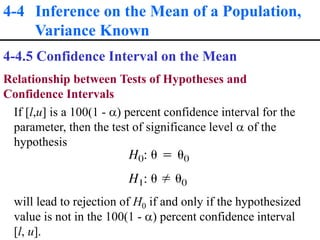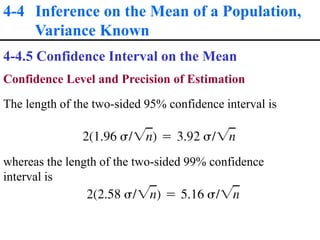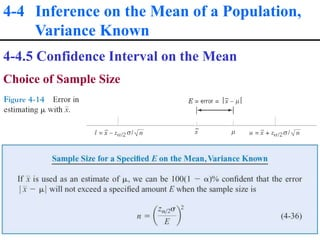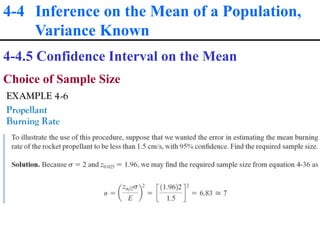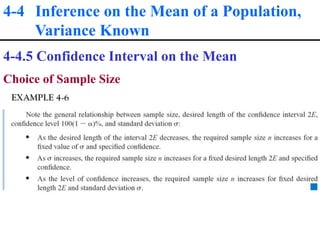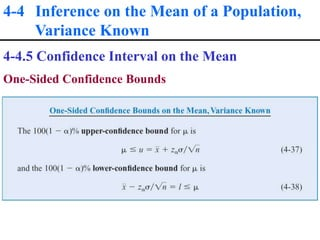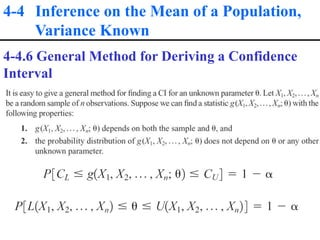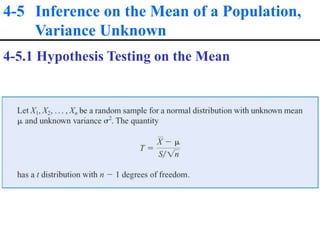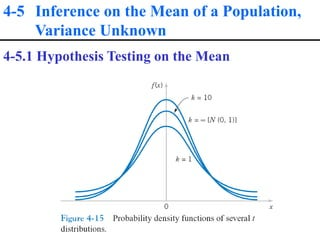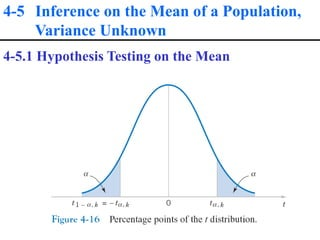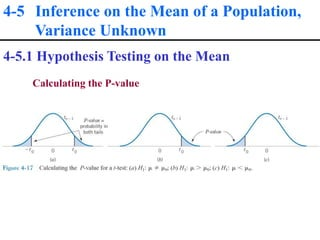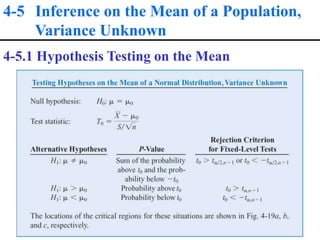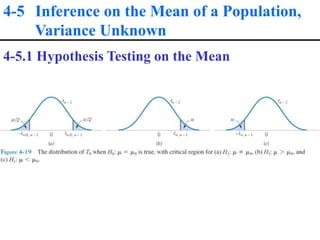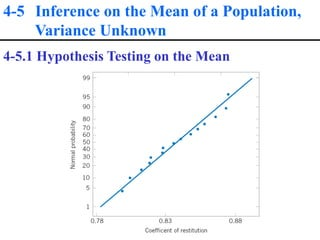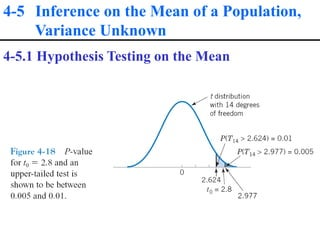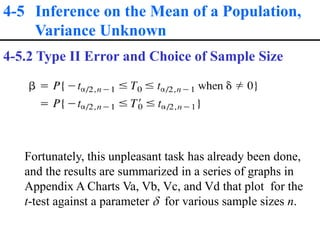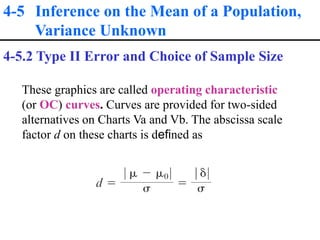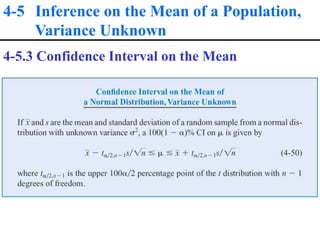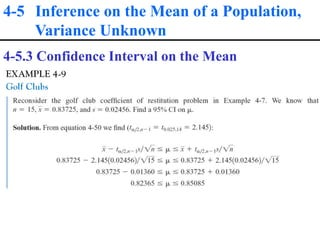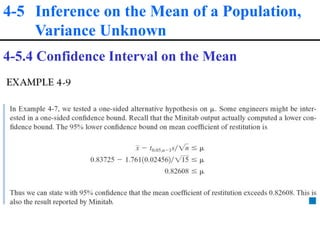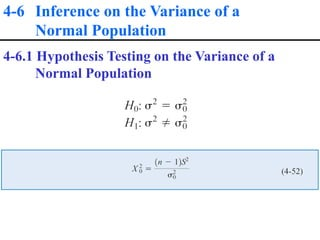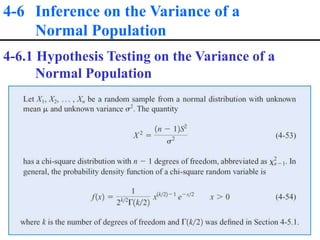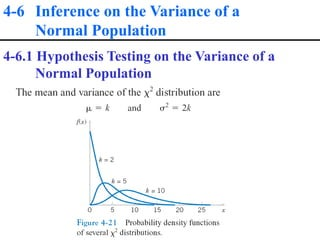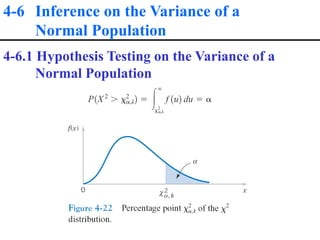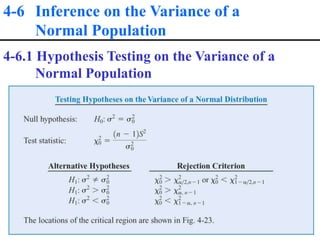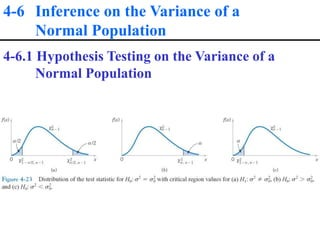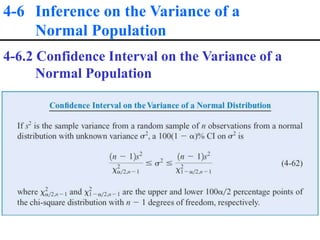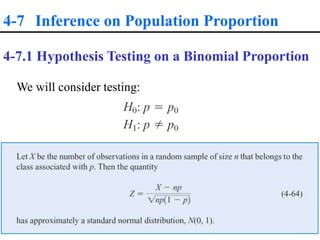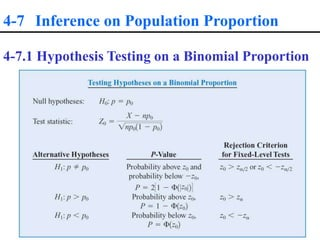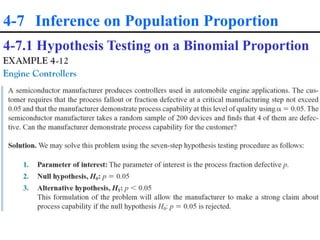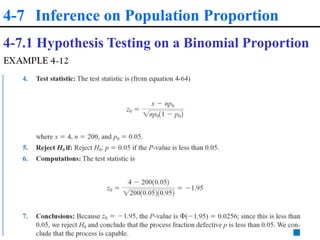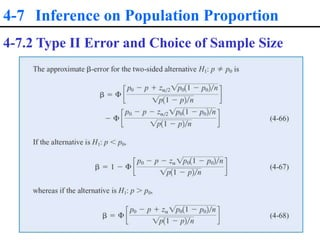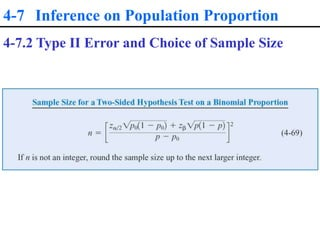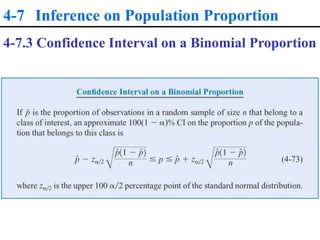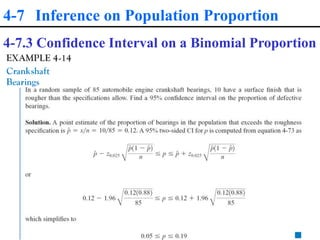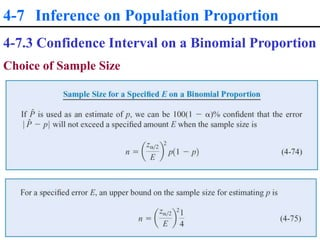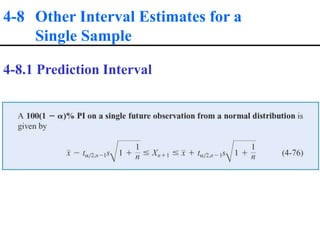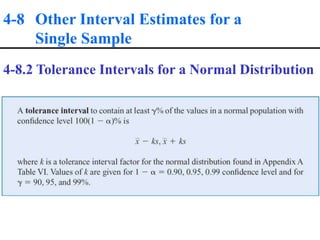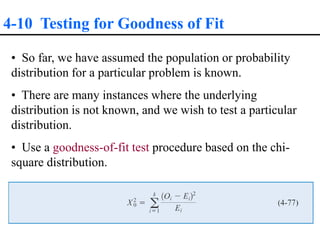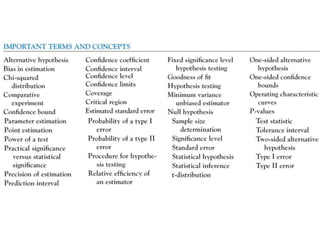1 of 82

### ch04.ppt

1. 4-1 Statistical Inference • The field of statistical inference consists of those methods used to make decisions or draw conclusions about a population. •These methods utilize the information contained in a sample from the population in drawing conclusions.
2. 4-1 Statistical Inference
3. 4-2 Point Estimation
4. 4-2 Point Estimation
5. 4-2 Point Estimation
6. 4-3 Hypothesis Testing We like to think of statistical hypothesis testing as the data analysis stage of a comparative experiment, in which the engineer is interested, for example, in comparing the mean of a population to a specified value (e.g. mean pull strength). 4-3.1 Statistical Hypotheses
7. 4-3 Hypothesis Testing For example, suppose that we are interested in the burning rate of a solid propellant used to power aircrew escape systems. • Now burning rate is a random variable that can be described by a probability distribution. • Suppose that our interest focuses on the mean burning rate (a parameter of this distribution). • Specifically, we are interested in deciding whether or not the mean burning rate is 50 centimeters per second. 4-3.1 Statistical Hypotheses
8. 4-3 Hypothesis Testing 4-3.1 Statistical Hypotheses Two-sided Alternative Hypothesis One-sided Alternative Hypotheses
9. 4-3 Hypothesis Testing 4-3.1 Statistical Hypotheses Test of a Hypothesis • A procedure leading to a decision about a particular hypothesis • Hypothesis-testing procedures rely on using the information in a random sample from the population of interest. • If this information is consistent with the hypothesis, then we will conclude that the hypothesis is true; if this information is inconsistent with the hypothesis, we will conclude that the hypothesis is false.
10. 4-3 Hypothesis Testing 4-3.2 Testing Statistical Hypotheses
11. 4-3 Hypothesis Testing 4-3.2 Testing Statistical Hypotheses
12. 4-3 Hypothesis Testing 4-3.2 Testing Statistical Hypotheses Sometimes the type I error probability is called the significance level, or the -error, or the size of the test.
13. 4-3 Hypothesis Testing 4-3.2 Testing Statistical Hypotheses
14. 4-3 Hypothesis Testing 4-3.2 Testing Statistical Hypotheses
15. 4-3 Hypothesis Testing 4-3.2 Testing Statistical Hypotheses
16. 4-3 Hypothesis Testing 4-3.2 Testing Statistical Hypotheses
17. 4-3 Hypothesis Testing 4-3.2 Testing Statistical Hypotheses
18. 4-3 Hypothesis Testing 4-3.2 Testing Statistical Hypotheses • The power is computed as 1 - b, and power can be interpreted as the probability of correctly rejecting a false null hypothesis. We often compare statistical tests by comparing their power properties. • For example, consider the propellant burning rate problem when we are testing H 0 : m = 50 centimeters per second against H 1 : m not equal 50 centimeters per second . Suppose that the true value of the mean is m = 52. When n = 10, we found that b = 0.2643, so the power of this test is 1 - b = 1 - 0.2643 = 0.7357 when m = 52.
19. 4-3 Hypothesis Testing 4-3.3 One-Sided and Two-Sided Hypotheses Two-Sided Test: One-Sided Tests:
20. 4-3 Hypothesis Testing 4-3.3 P-Values in Hypothesis Testing
21. 4-3 Hypothesis Testing 4-3.5 General Procedure for Hypothesis Testing
22. 4-4 Inference on the Mean of a Population, Variance Known Assumptions
23. 4-4 Inference on the Mean of a Population, Variance Known 4-4.1 Hypothesis Testing on the Mean We wish to test: The test statistic is:
24. 4-4 Inference on the Mean of a Population, Variance Known 4-4.1 Hypothesis Testing on the Mean Reject H0 if the observed value of the test statistic z0 is either: or Fail to reject H0 if
25. 4-4 Inference on the Mean of a Population, Variance Known 4-4.1 Hypothesis Testing on the Mean
26. 4-4 Inference on the Mean of a Population, Variance Known 4-4.1 Hypothesis Testing on the Mean
27. 4-4 Inference on the Mean of a Population, Variance Known 4-4.2 Type II Error and Choice of Sample Size Finding The Probability of Type II Error b
28. 4-4 Inference on the Mean of a Population, Variance Known 4-4.2 Type II Error and Choice of Sample Size Sample Size Formulas
29. 4-4 Inference on the Mean of a Population, Variance Known 4-4.2 Type II Error and Choice of Sample Size Sample Size Formulas
30. 4-4 Inference on the Mean of a Population, Variance Known 4-4.2 Type II Error and Choice of Sample Size
31. 4-4 Inference on the Mean of a Population, Variance Known 4-4.2 Type II Error and Choice of Sample Size
32. 4-4 Inference on the Mean of a Population, Variance Known 4-4.3 Large Sample Test In general, if n  30, the sample variance s2 will be close to σ2 for most samples, and so s can be substituted for σ in the test procedures with little harmful effect.
33. 4-4 Inference on the Mean of a Population, Variance Known 4-4.4 Some Practical Comments on Hypothesis Testing The Seven-Step Procedure Only three steps are really required:
34. 4-4 Inference on the Mean of a Population, Variance Known 4-4.4 Some Practical Comments on Hypothesis Testing Statistical versus Practical Significance
35. 4-4 Inference on the Mean of a Population, Variance Known 4-4.4 Some Practical Comments on Hypothesis Testing Statistical versus Practical Significance
36. 4-4 Inference on the Mean of a Population, Variance Known 4-4.5 Confidence Interval on the Mean Two-sided confidence interval: One-sided confidence intervals: Confidence coefficient:
37. 4-4 Inference on the Mean of a Population, Variance Known 4-4.5 Confidence Interval on the Mean
38. 4-4 Inference on the Mean of a Population, Variance Known 4-4.6 Confidence Interval on the Mean
39. 4-4 Inference on the Mean of a Population, Variance Known 4-4.5 Confidence Interval on the Mean
40. 4-4 Inference on the Mean of a Population, Variance Known 4-4.5 Confidence Interval on the Mean Relationship between Tests of Hypotheses and Confidence Intervals If [l,u] is a 100(1 - ) percent confidence interval for the parameter, then the test of significance level  of the hypothesis will lead to rejection of H0 if and only if the hypothesized value is not in the 100(1 - ) percent confidence interval [l, u].
41. 4-4 Inference on the Mean of a Population, Variance Known 4-4.5 Confidence Interval on the Mean Confidence Level and Precision of Estimation The length of the two-sided 95% confidence interval is whereas the length of the two-sided 99% confidence interval is
42. 4-4 Inference on the Mean of a Population, Variance Known 4-4.5 Confidence Interval on the Mean Choice of Sample Size
43. 4-4 Inference on the Mean of a Population, Variance Known 4-4.5 Confidence Interval on the Mean Choice of Sample Size
44. 4-4 Inference on the Mean of a Population, Variance Known 4-4.5 Confidence Interval on the Mean Choice of Sample Size
45. 4-4 Inference on the Mean of a Population, Variance Known 4-4.5 Confidence Interval on the Mean One-Sided Confidence Bounds
46. 4-4 Inference on the Mean of a Population, Variance Known 4-4.6 General Method for Deriving a Confidence Interval
47. 4-5 Inference on the Mean of a Population, Variance Unknown 4-5.1 Hypothesis Testing on the Mean
48. 4-5 Inference on the Mean of a Population, Variance Unknown 4-5.1 Hypothesis Testing on the Mean
49. 4-5 Inference on the Mean of a Population, Variance Unknown 4-5.1 Hypothesis Testing on the Mean
50. 4-5 Inference on the Mean of a Population, Variance Unknown 4-5.1 Hypothesis Testing on the Mean Calculating the P-value
51. 4-5 Inference on the Mean of a Population, Variance Unknown 4-5.1 Hypothesis Testing on the Mean
52. 4-5 Inference on the Mean of a Population, Variance Unknown 4-5.1 Hypothesis Testing on the Mean
53. 4-5 Inference on the Mean of a Population, Variance Unknown 4-5.1 Hypothesis Testing on the Mean
54. 4-5 Inference on the Mean of a Population, Variance Unknown 4-5.1 Hypothesis Testing on the Mean
55. 4-5 Inference on the Mean of a Population, Variance Unknown 4-5.2 Type II Error and Choice of Sample Size Fortunately, this unpleasant task has already been done, and the results are summarized in a series of graphs in Appendix A Charts Va, Vb, Vc, and Vd that plot for the t-test against a parameter d for various sample sizes n.
56. 4-5 Inference on the Mean of a Population, Variance Unknown 4-5.2 Type II Error and Choice of Sample Size These graphics are called operating characteristic (or OC) curves. Curves are provided for two-sided alternatives on Charts Va and Vb. The abscissa scale factor d on these charts is defined as
57. 4-5 Inference on the Mean of a Population, Variance Unknown 4-5.3 Confidence Interval on the Mean
58. 4-5 Inference on the Mean of a Population, Variance Unknown 4-5.3 Confidence Interval on the Mean
59. 4-5 Inference on the Mean of a Population, Variance Unknown 4-5.4 Confidence Interval on the Mean
60. 4-6 Inference on the Variance of a Normal Population 4-6.1 Hypothesis Testing on the Variance of a Normal Population
61. 4-6 Inference on the Variance of a Normal Population 4-6.1 Hypothesis Testing on the Variance of a Normal Population
62. 4-6 Inference on the Variance of a Normal Population 4-6.1 Hypothesis Testing on the Variance of a Normal Population
63. 4-6 Inference on the Variance of a Normal Population 4-6.1 Hypothesis Testing on the Variance of a Normal Population
64. 4-6 Inference on the Variance of a Normal Population 4-6.1 Hypothesis Testing on the Variance of a Normal Population
65. 4-6 Inference on the Variance of a Normal Population 4-6.1 Hypothesis Testing on the Variance of a Normal Population
66. 4-6 Inference on the Variance of a Normal Population 4-6.2 Confidence Interval on the Variance of a Normal Population
67. 4-7 Inference on Population Proportion 4-7.1 Hypothesis Testing on a Binomial Proportion We will consider testing:
68. 4-7 Inference on Population Proportion 4-7.1 Hypothesis Testing on a Binomial Proportion
69. 4-7 Inference on Population Proportion 4-7.1 Hypothesis Testing on a Binomial Proportion
70. 4-7 Inference on Population Proportion 4-7.1 Hypothesis Testing on a Binomial Proportion
71. 4-7 Inference on Population Proportion 4-7.2 Type II Error and Choice of Sample Size
72. 4-7 Inference on Population Proportion 4-7.2 Type II Error and Choice of Sample Size
73. 4-7 Inference on Population Proportion 4-7.3 Confidence Interval on a Binomial Proportion
74. 4-7 Inference on Population Proportion 4-7.3 Confidence Interval on a Binomial Proportion
75. 4-7 Inference on Population Proportion 4-7.3 Confidence Interval on a Binomial Proportion Choice of Sample Size
76. 4-8 Other Interval Estimates for a Single Sample 4-8.1 Prediction Interval
77. 4-8 Other Interval Estimates for a Single Sample 4-8.2 Tolerance Intervals for a Normal Distribution
78. 4-10 Testing for Goodness of Fit • So far, we have assumed the population or probability distribution for a particular problem is known. • There are many instances where the underlying distribution is not known, and we wish to test a particular distribution. • Use a goodness-of-fit test procedure based on the chi- square distribution.